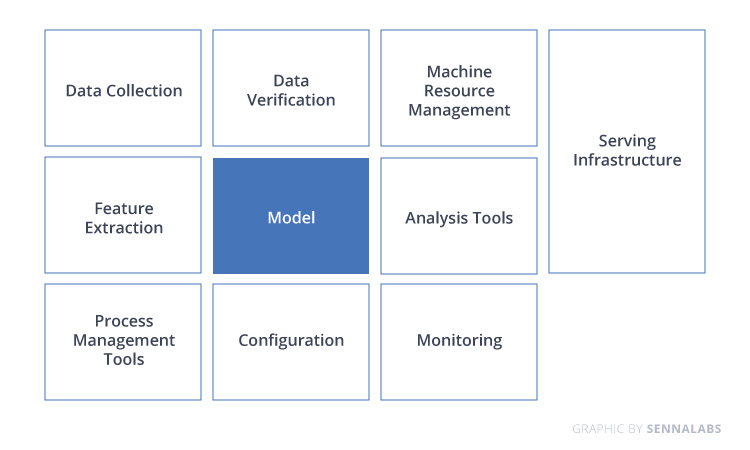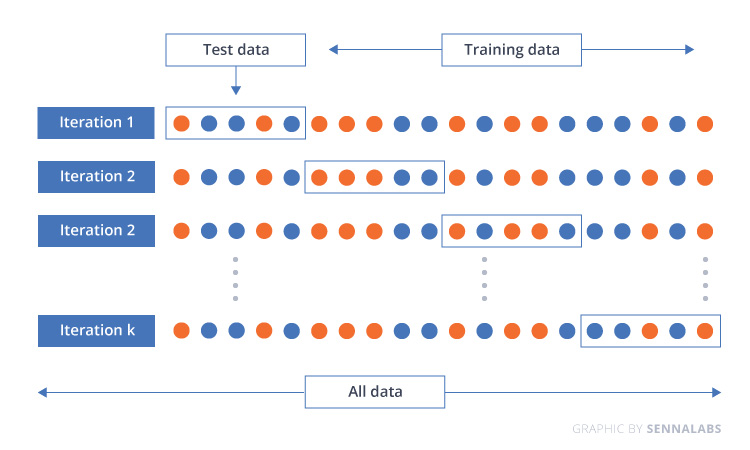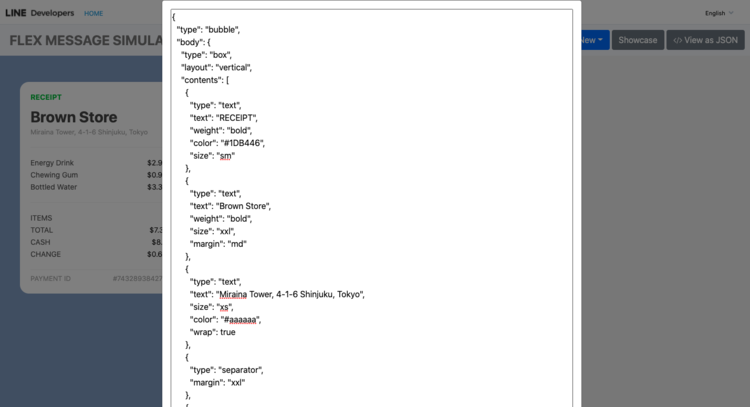15Sep, 2020
Language blog :
English
Share blog :
15 September, 2020
English

# How to perform Quality Assurance for Machine Learning models

ByMachine learning is a method of data analysis that automates analytical model building. It is a branch of artificial intelligence based on the idea that systems can learn from data, identify patterns, and make decisions with minimal human intervention. Machine learning eliminates the need to provide explicit instructions for the systems to function on a regular basis.

Traditional ML methodology has focused more on the model development process, which involved selecting the most appropriate algorithm for a given problem. In contrast, the software development process focuses on both the development and testing of the software.ML development pipeline

Testing about ML model development focuses more on the model's performance in terms of its accuracy. This activity is generally carried out by the data scientist developing the model. These models have significant real-world implications as they are crucial for decision making at the highest level.

## Model Evaluation Techniques

Methods for evaluating a model's performance are divided into two categories: holdout and Cross-validation. Both methods use a test set (data not seen by the model) to evaluate model performance. It is not recommended to use the data we used to build the model to evaluate it. Since our model will remember the whole training set and always predict the correct label for any point in the training set, which is known as overfitting.

### Holdout

• The training set is a subset of the dataset used to build predictive models.
• The validation set is a subset of the dataset used to assess the performance of the model built in the training phase. It provides a test platform for fine-tuning a model's parameters and selecting the best performing model. Not all modeling algorithms need a validation set.
• The test set, or unseen data, is a subset of the dataset used to assess the likely future performance. If a model fits the training set much better than it does the test set, overfitting is probably the cause.

### Cross-Validation

The most common cross-validation technique is "k-fold cross-validation". In this technique, the original dataset is partitioned into k equal-sized subsamples, called "folds." The k is a user-specified number, usually with 5 or 10 as its preferred value. This is repeated k times, such that each time, one of the k subsets is used as the test set/validation set, and the other k-1 subsets are put together to form a training set. The error estimation is averaged over all k trials to get the total effectiveness of our model.## Model Evaluation Metrics

Model evaluation metrics are required to quantify model performance. The choice of evaluation metrics depends on a given machine learning task (such as classification, regression, ranking, clustering, topic modeling, among others).

### Classification Accuracy

Accuracy is a standard evaluation metric for classification problems. It's the number of correct predictions made as a ratio of all predictions made. By using cross-validation, we'd be "testing" our machine learning model in the "training" phase to check for overfitting and to get an idea about how our machine learning model will generalize to independent data (test data set).

### Confusion Matrix

The diagonal elements represent the number of points for which the predicted label is equal to the true label, while the classifier mislabeled anything off the diagonal. Therefore, the higher the diagonal values of the confusion matrix, the better, indicating many correct predictions.### Logarithmic Loss

Logarithmic loss (log loss) measures a classification model's performance where the prediction input is a probability value between 0 and 1. Log loss increases as the predicted probability diverge from the actual label. The goal of machine learning models is to minimize this value. As such, smaller log loss is better, with a perfect model having a log loss of 0.

### F-measure

F-measure (also F-score) is a measure of a test's accuracy that considers both the precision and the test's recall to compute the score. Precision is the number of correct positive results divided by the total predicted positive observations. On the other hand, recall is the number of correct positive results divided by the number of all relevant samples (total actual positives).## Conclusion

The estimated performance of a model tells us how well it performs on unseen data. Making predictions on future data is often the main problem we want to solve. It is essential to understand the context before choosing a metric because each machine learning model tries to solve a problem with a different objective using a different dataset.

_____

Reference:

Written bySenna Labs- More than 120,000 people/day visit to read our blogs

## Other articles for you

29
September, 202329 September, 2023
Explanation of different kinds of Machine Learning models/strategies and their use cases
Last time, we mentioned how to invest a machine learning for an MVP product successfully. In this article, we will go furthermore on how to choose an appropriate machine learning

By

English
29
September, 202329 September, 2023
Choosing the appropriate machine algorithm in real use cases
In the real machine learning project, a typical question that always asked is; when facing a wide variety of machine algorithm, is "Which algorithm should we use ?" but the

By

English
29
September, 202329 September, 2023
How to successfully invest in machine learning in an MVP
A minimum viable product (MVP) is a version of a product with contains enough features to satisfy early customers and validate ideas early in the development cycle for future development.

By

English

## Let’s build digitalproductsthat aresimply awesome !

We will get back to you within 24 hours!Please tell us your ideas.
- Senna Labsmake it happy28/11 Soi Ruamrudee, Lumphini, Pathumwan, Bangkok 10330+66 62 389 4599hello@sennalabs.com© 2022 Senna Labs Co., Ltd.All rights reserved.